WizardJoined: Oct 14, 2009
• Posts: 25135
October 20th, 2022 at 7:56:28 AM permalink
In episode 4 of Survivor season 43, which I believe aired on Oct. 12, 2022, there was a geometric challenge. Each team had a bag of four puzzle pieces. First, they had to arrange the pieces to construct a square. Then they had to move them to another area and construct an equilateral triangle.

This is known as The Haberdasher's Puzzle/Problem

Here is the original footage:

part 1
part 2
part 3

Here is a picture of the pieces forming a square: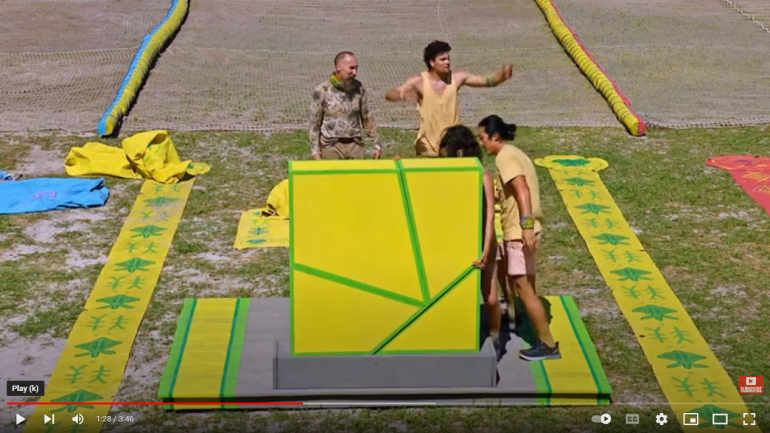Here is the triangle.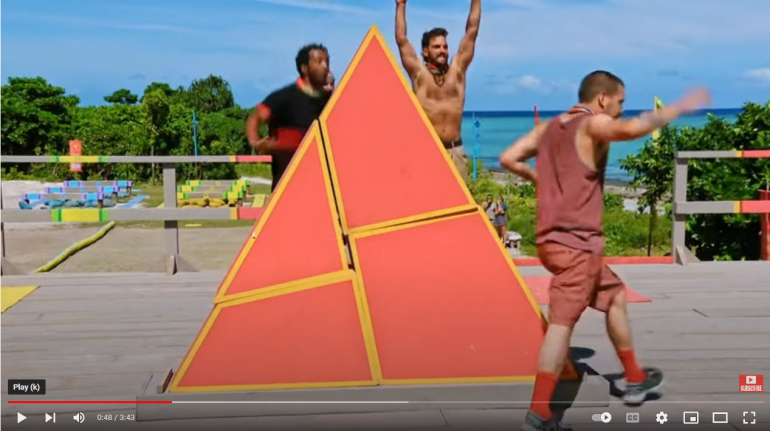My question for the thread is what are the dimensions of the four pieces? Please assume the square has sides of length 1.

I have already worked on this a fair bit and have an answer. It's possible there are an infinite number of answers.
Last edited by: Wizard on Oct 20, 2022
�Extraordinary claims require extraordinary evidence.� -- Carl Sagan
WizardJoined: Oct 14, 2009
• Posts: 25135
October 20th, 2022 at 8:21:52 PM permalink
I think I'm talking to myself in this thread, but I believe I have a firm answer.
�Extraordinary claims require extraordinary evidence.� -- Carl Sagan
chevyJoined: Apr 15, 2011
• Posts: 115
October 20th, 2022 at 11:09:50 PM permalink
Well, if there are more than one, here is maybe one

The Area of the triangle = Area of square = 1^2 = 1

For equilateral triangle of side S, will have height sqrt(3)/2 * S and area = (1/2)*(S/2)*(sqrt(3)/2 * S)

So I get side of triangle S = 8/sqrt(3)

In my drawing,
a) the two crossing lines are perpendicular. Both are length = 1 (side of of square) and A1=A2=A3=A4 = 1/2
b) the crossing lines are symmetric, so dotted red line makes an equilateral triangle of side B = sqrt(2)/2
c) So C = S-B = 8/sqrt(3) - sqrt(2)/2
d) E = B again by symmetry
e) D = .5*(S-E) = .5*(S-B) = .5*C = .5 *(8/sqrt(3) - sqrt(2)/2)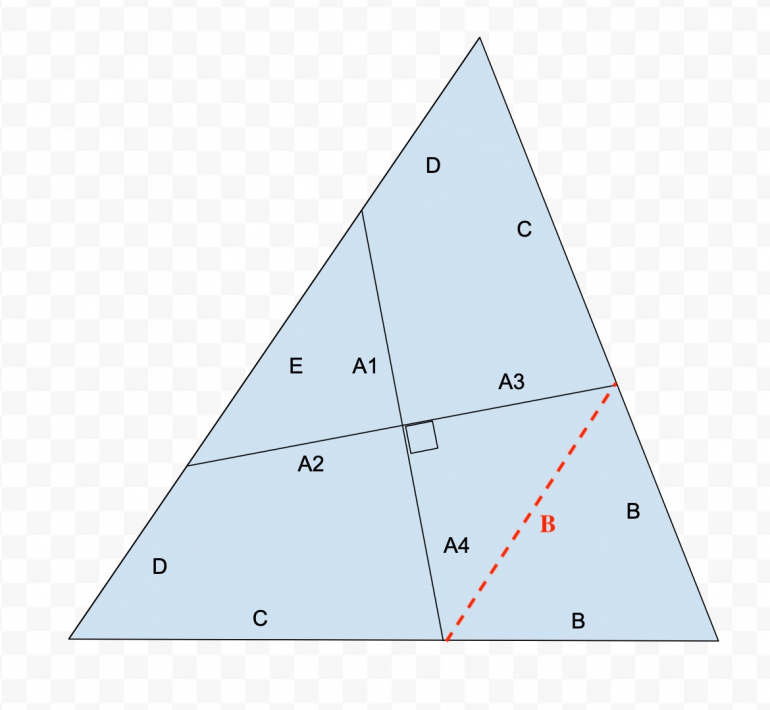The needed conditions I think in general are that
i) A1+A2 =1 (side of triangle) and they are perpendicular
ii) A3+A4 =1 (side of triangle) and they are perpendicular

On original pic I labelled how they would be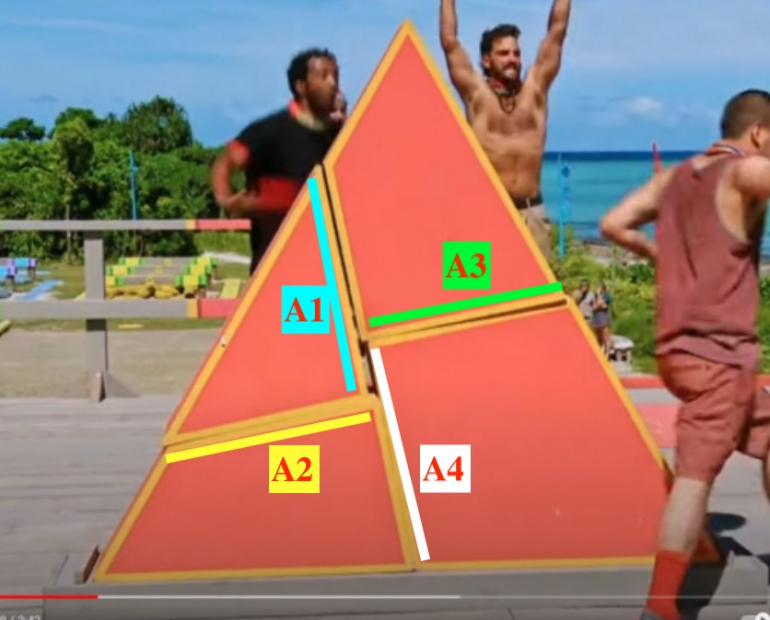Anyway, that is a shot.....may have done my trig, Pythagorean theorem, or algebra wrong.....but art least you aren't talking to yourself?
TheCapitalShipJoined: Aug 14, 2021
• Posts: 40
October 20th, 2022 at 11:18:04 PM permalink
I am intrigued by this, geometry is sadly not my strong suit. But I will take a stab at this tommorow...I will post my answer if I get somewhere.
chevyJoined: Apr 15, 2011
• Posts: 115
October 21st, 2022 at 6:55:29 AM permalink
Current opinion.......My above construction fails

I was only looking at the interior crosses and needing right angles and two legs adding to give side of square.

BUT.....I also need the pieces to fit together to form square. in my picture that would mean B=C....and sadly that does not work.

Will think about it more if I have the time
WizardJoined: Oct 14, 2009
• Posts: 25135
October 21st, 2022 at 7:07:10 AM permalink
Quote: chevy

Current opinion.......My above construction fails

I'm glad to see interest in this problem.

I too did it your way at first and accused the triangle on Survivor of not being a true equilateral triangle. However, I later found an error in my work and deleted the accusation.

In your diagram you have D twice, indicating those positions are the same length. My solution does not have them equal. Close though.

For the actual dimensions of the pieces, I ask for only a handful of decimal places, like six. I think to put them in closed form would be too much to ask.
�Extraordinary claims require extraordinary evidence.� -- Carl Sagan
chevyJoined: Apr 15, 2011
• Posts: 115
October 21st, 2022 at 2:15:38 PM permalink
My second (and probably final attempt)

Here it is in picture form.

I got two values for E&F (per Wizard's comment.....) Close but different. I assigned the longer one to the segment that I thought had to be longer, but can't conclusively prove it....so they could be switched.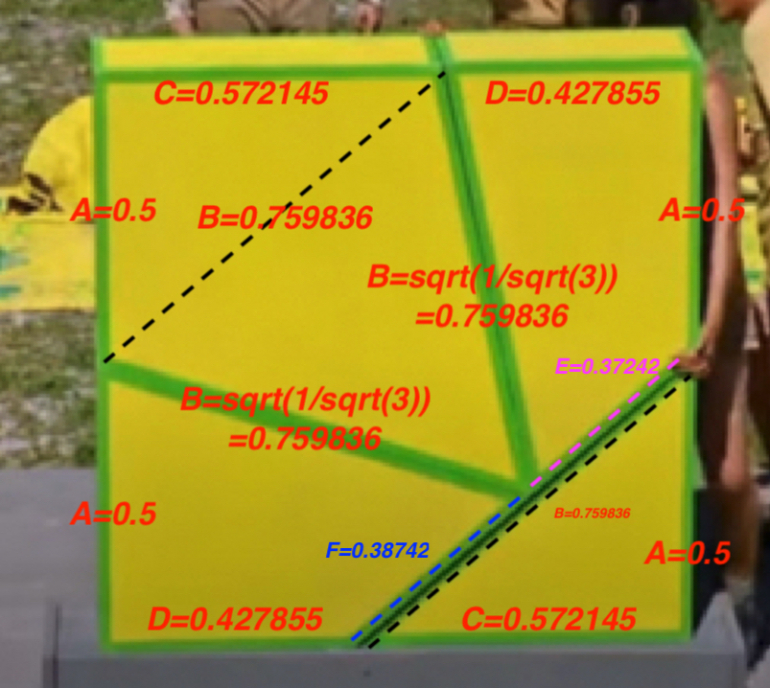TheCapitalShipJoined: Aug 14, 2021
• Posts: 40
October 21st, 2022 at 8:01:59 PM permalink
Quote: chevy

My second (and probably final attempt)

Here it is in picture form.

I got two values for E&F (per Wizard's comment.....) Close but different. I assigned the longer one to the segment that I thought had to be longer, but can't conclusively prove it....so they could be switched.I got the same value for B, my question to you is, on the kite piece, we know the two long sides are the same length, and that the adjacent angle is 60 degrees (since it's used as a corner on the triangle), wouldn't that mean it's an equilateral triangle? Which means that would make triangle ABC a 45, 45, 90 triangle? Or am I missing an extra step in there somewhere?
WizardJoined: Oct 14, 2009
• Posts: 25135
October 21st, 2022 at 8:45:02 PM permalink
Quote: chevy

My second (and probably final attempt)

Here it is in picture form.

I got two values for E&F (per Wizard's comment.....) Close but different. I assigned the longer one to the segment that I thought had to be longer, but can't conclusively prove it....so they could be switched.I agree!
�Extraordinary claims require extraordinary evidence.� -- Carl Sagan
chevyJoined: Apr 15, 2011
• Posts: 115
October 21st, 2022 at 8:49:43 PM permalink
Quote: TheCapitalShip

Quote: chevy

My second (and probably final attempt)

....clipped and moved to my reply....

TheCapitalShip: "I got the same value for B, my question to you is, on the kite piece, we know the two long sides are the same length, and that the adjacent angle is 60 degrees (since it's used as a corner on the triangle), wouldn't that mean it's an equilateral triangle? Which means that would make triangle ABC a 45, 45, 90 triangle? Or am I missing an extra step in there somewhere?"
"

Yes, I got that the "bottom of the kite" is a B-B-B equilateral triangle. (Third "B" is the dashed line)

Then to get C = sqrt (B^2 - A^2).....since ABC is right triangle and pythagorean theorem and we know B and A***

So the numbers don't end up as 45-45-90.

***You didn't mention it, but did you get A = 0.5? I used that fact in finding C. The top of left side is A and the bottom of the left side is A since they both come from the same cut on the triangle view. So each must be 0.5 (square side is 1)
Same for the right side by the way.

From this view, I used
ALB=A on Left Bottom of my square
ALT=A on Left Top of my square
ARB=A on Right Bottom of my square
ART=A on Right Top of my square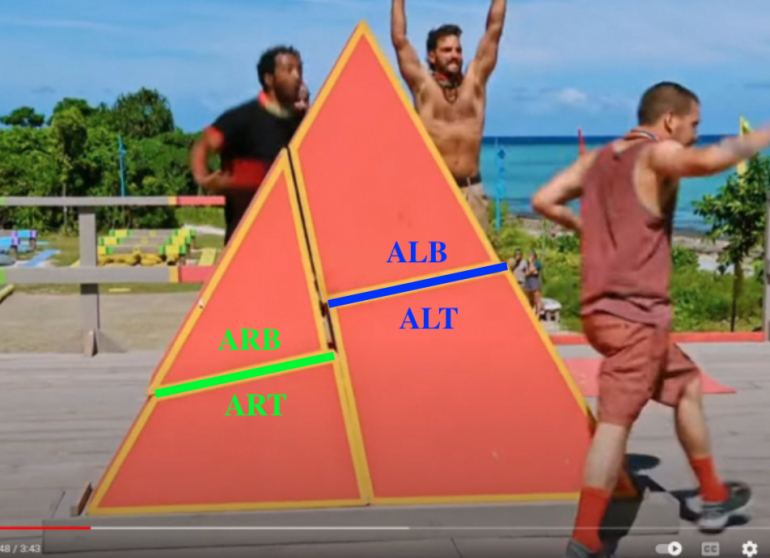PS: As I see it, all the pieces in the survivor yellow team's square are the OPPOSITE side view of the survivor red team's triangle..i.e. we are seeing the construction from two different sides. (...easiest to see looking at the long side of the triangle piece.)